﻿ Modeling of Dynamic Systems in Simulation Environment MATLAB/Simulink – SimMechanics

Modeling of Dynamic Systems in Simulation Environment MATLAB/Simulink – SimMechanics

Peter Frankovský, Darina Hroncová, Ingrid Delyová, Ivan VirgalaOPEN ACCESSPEER-REVIEWED

Modeling of Dynamic Systems in Simulation Environment MATLAB/Simulink – SimMechanics

Peter Frankovský1,, Darina Hroncová1, Ingrid Delyová1, Ivan Virgala1

1Department of Applied Mechanics and Mechatronics, Faculty of Mechanical Engineering, Technical University of Košice, Košice, Slovakia

Abstract

The work shows the use of SimMechanics program for modeling of mechanical systems. The presented paper aims to determination the responses of the mechanical vibrating system through non-homogeneous linear differential equation of second order with constant coefficients using MATLAB/Simulink and SimMechanics. SimMechanics formulates and solves the equations of motion of mechanical system.

At a glance: Figures

1234567
Prev Next

• Frankovský, Peter, et al. "Modeling of Dynamic Systems in Simulation Environment MATLAB/Simulink – SimMechanics." American Journal of Mechanical Engineering 1.7 (2013): 282-288.
• Frankovský, P. , Hroncová, D. , Delyová, I. , & Virgala, I. (2013). Modeling of Dynamic Systems in Simulation Environment MATLAB/Simulink – SimMechanics. American Journal of Mechanical Engineering, 1(7), 282-288.
• Frankovský, Peter, Darina Hroncová, Ingrid Delyová, and Ivan Virgala. "Modeling of Dynamic Systems in Simulation Environment MATLAB/Simulink – SimMechanics." American Journal of Mechanical Engineering 1, no. 7 (2013): 282-288.

 Import into BibTeX Import into EndNote Import into RefMan Import into RefWorks

1. Introduction

Simulation of mechanical systems with more degrees of freedom is a common issue in engineering. There are various software applications available to deal with the issue, which use either symbolic equations or, based on a model, adopt numerical solution by means of rather more abstract representation such as block diagrams. SimMechanics, designed as an extension to program MATLAB, falls within the second-mentioned category.

As an extension to MATLAB/Simulink, SimMechanics enriches the features of Simulink through the implementation of additional tools to model and simulate mechanical systems. It includes block libraries which represent actual parts of mechanical systems. There are blocks which represent bodies, knuckle joints, endless screws, dampers, springs, sensors and actuators. These blocks enable us to design models of complex mechanical systems.

In order to solve equations of motion, the program MATLAB directly uses functions for numerical solution of differential equations. Similarly to Simulink, which is a program part of MATLAB, the extension application SimMechanics handles mechanical systems by means of block diagrams. Block diagrams in SimMechanics are direct representations of physical components (e.g. springs and dampers), geometric and kinematic relations, what differs them from block diagrams in Simulink. Contrary to the above-mentioned, the blocks in Simulink represent mathematical operations. On that account, physical modeling in the extension SimMechanics is more intuitive, less time-consuming and requires less effort when deriving equations of motion of particular mechanical system [1, 3, 4].

Within its models though, SimMechanics still enables us to use blocks from the Simulink environment. In this way it is possible to design a mechanical model in SimMechanics and, simultaneously, incorporate a control part of Simulink into one common environment [3, 4].

2. Model of the Mechanical System with One Degree of Freedom

The mechanical system with one degree of freedom subject to the analysis is a body with mass m fixed to a solid frame Figure 1 with a spring and a damper. The mass of the spring is not considered. The system is excited harmonically by variable force F (t) and moves linearly in the direction of spring axis and damper axis . A harmonically variable force F(t) = F0sin(ωt) is used for the model excitation.

Dynamic equation of motion which applies to the mechanical system Figure 1 in its vector form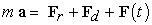(1)

Substituting the forces equation of the motion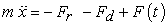(2)

where m - the mass of the body,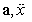- acceleration of the mass,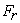- reaction force in the spring,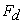- damping force,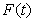- excitation force.

The reaction force in the spring is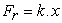(3)

The damping force is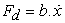(4)

The excitation force is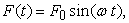(5)

Where k - spring constant,

b - viscous damping coefficient,

x – displacement of the spring,

ω – natural frequency of the exciting force.

After substitution of relation (3), (4), (5) to the equation (2) we receive the acceleration of mechanical system with one degree of freedom as follows: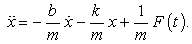(6)

The derived equation of motion (6) is further solved in the program MATLAB. As a result we gain kinematic values (displacement, velocity and acceleration) of the mechanical system in relation to time t.

3. Solution of the Mechanical System with One Degree of Freedom in MATLAB

In MATLAB the derived non-homogenous linear differential equation of second order with constant coefficients (6) was solved in relation to mechanical system with one degree of freedom by means of Runge-Kutta method. The following parameters were taken into consideration:

- mass m=1 [kg],

- viscous damping coefficient b=0 [N/(m/s)],

- spring constant k=(2 π)2 [N/m],

- exciting force F(t)= F0.sin(ωt), F0=1 [N],

considering three different frequencies of exciting force:

- natural frequency of exciting force ω=1,

- natural frequency of exciting force ω=π,

- natural frequency of exciting force

ω=√(k/m)=√((2π)2/1)=2π.

The following conditions are initial conditions for the solution of mechanical system:

- x(0)=0 [m],

- v(0)=0 [m/s],

with solution period 0 ≤ t ≤ 20.

Prior to solution in MATLAB it is needed to create two m-files. The first is the m-function-file defining the language of MATLAB while the second m-file shall specify initial conditions and the integration period.

The m-files have the following form:

functionTDS_F.m

function dx=function TDS_F(t,x)

m=1; k=(2*pi)^2; b=0;

F0=1;

omega=pi;

F=F0*sin(omega*t);

dx=[x(2); -k * x(1) / m - b * x(2) / m + F/m];

vibration.m

[t,x]=ode45(@functionTDS_F,[0 20],[0.0;0.0]);

% [0 20] – parameters of time simulation (start time, end time), [0;0] – initial conditions (displacement, velocity)

% ode45 – numerical solution of differential equations systems, application of Runge-Kutta method of fourth order

plot(t,x)

title('Kinematic variables x(t),v(t) in MATLAB: F=sin(\omega*t), \omega = \pi ');

xlabel('t [s]');

ylabel('x [m], v [m/s]');

grid on;

legend('x=x(t)','v=v(t)');

Results of displacements and velocity of the mechanical system in relation to frequencies of exciting force ω=1, ω=π, ω=√(k/m)=√((2π)2/1)=2π in relation to time t are shown in Figure 2, Figure 3 and Figure 4 by means of function plot(t, x).

4. Solution of the Mechanical System with One Degree of Freedom in SimMechanics

SimMechanics is an extension to Simulink which was designed for modeling of systems with more degrees of freedom. It includes a library of blocks which constitute real parts of the mechanism (e.g. bodies and connections between them, actuators, sensors etc.). These blocks are in later stages processed on physical level. The model of actual technical object is designed when combining these blocks. Connecting physical blocks together a mathematical model is automatically designed for solution in Simulink. This method enables us to solve complex tasks in case of which manual derivation of equations of motion would be time-consuming or would cost much effort or might not be possible at all.

The calculation of kinematical values of mechanical system with one degree of freedom shall be done in SimMechanics for the same parameters of mechanical system with one degree of freedom as in case of kinematical values in MATLAB. In SimMechanics we design a block diagram in Figure 5 based on body geometry and connections regarding the environment in question. The block diagram shall further be processed in Simulink.

Simulation results of kinematical parameters of mechanical system (displacement, velocity acceleration) are shown in Figure 6 to Figure 8 by means of plot function of m-file:

% variables simout in Workspace– results parameters x(t),v(t),a(t) after processing in SimMechanics

Figure 3

set(3,'Name','results x(t), v(t), a(t) in SimMechanics')

plot(simout.time, simout.signals.values(:,1:3), 'LineWidth', 2)

title('SimMechanics:x=x(t),v(t),a=a(t): m=1kg,F0=1,k=(2*pi)^2,\omega=\pi'),

legend('x=x(t)','v=v(t)','a=a(t)'),

xlabel('t [s]'),

ylabel('x [m], v [m/s], a [m/s^2]'),

grid on

Figure 6. Simulation results of displacement x(t),velocity v(t) and acceleration a(t) in relation to frequency of exciting force ω=1
Figure 7. Simulation results of displacement x(t),velocity v(t) and acceleration a(t) in relation to natural frequency of exciting force ω= π
Figure 8. Simulation results of displacement x(t),velocity v(t) and acceleration a(t) in relation to natural frequency of exciting force ω=2π

Simulation results of kinematical values (displacement, velocity) are shown in Figure 9, Figure 10, Figure 11 by means of plot function of m-file:

% variables simout in Workspace – results parameters x(t),v(t) after processing in SimMechanics

set(2,'Name','results x(t), and v(t) in SimMechanics')

plot(simout.time, simout.signals.values(:,1:2), 'LineWidth', 2)

title('SimMechanics:x=x(t),v(t),m=1kg,F0=1,k=(2*pi)^2, \omega=\pi'),

legend('x=x(t)','v=v(t)'),

xlabel('t [s]'),

ylabel('x [m], v [m/s]'),

grid on

As expected, the results of solution in SimMechanics in Figure 9, Figure 10, Figure 11 are equal to solution in MATLAB Figure 2, Figure 3, Figure 4.

Figure 9. Simulation results of displacement x(t) and velocity v(t) in SimMechanics – natural frequency of exciting force ω=1
Figure 10. Simulation results of displacement x(t) and velocity v(t) in SimMechanics with natural frequency of exciting force ω= π
Figure 11. Simulation results of displacement x(t) and velocity v(t) in SimMechanics with natural frequency of exciting force ω= 2π

In the following stage the courses of displacements x(t) for frequencies of exciting force are depicted in an analogous way: ω=1, ω=π, ω=2π in Figure 12, Figure 13, Figure 14.

5. Mechanical System with Two Degrees of Freedom

In the following part of the paper we present a model representing mechanical system with two degrees of freedom as solved in MATLAB and SimMechanics. The model consists of two bodies with masses m1 and m2 which are fixed to a solid frame with a spring of spring constant k1 and a damper with linear damping coefficient b1. The bodies are bound to each other with a spring with spring constant k2 and a damper with linear damping coefficient b2. Masses of the springs shall be ignored. The mass m1 is excited by external harmonic force F(t) = F0sin(ωt) in the axis direction of the spring and the damper, where F0=konst. The output of the solution the model of mechanical system in Figure 15 in MATLAB and SimMechanics are simulation results of displacements, velocities and accelerations of individual system members.

Differential second order equations of motions related to the mechanical system depicted in Figure 15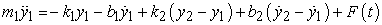(7)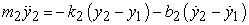(8)

shall be processed in order to be solved in MATLAB and shall gain the form of first order differential equations through the following substitution: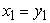(9)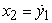(10)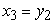(11)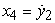(12)

while transforming second order differential equations (7) and (8) to four first order differential equations as follows: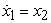(13)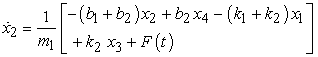(14)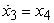(15)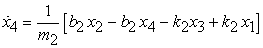(16)

After the above-described processing the set of equations are rewritten into the m-file of MATLAB in the following form:

function dx=functionTDS_2(t,x)

m1=75; m2=150; k1=500; k2=250;

b1=10; b2=50; F=100*sin(2*t);

dx=[x(2); ...

(-(b1 + b2) * x(2) + b2 * x(4) - (k1 + k2)*x(1)+ ...

k2*x(3) + F)/m1; ...

x(4); ...

(b2*x(2)-b2*x(4)-k2*x(3)+k2*x(1))/m2];

MATLAB includes predefined functions, which differ in relevant integration method, to solve differential equations (7) and (8) numerically. The solution of required kinematic values shall be done after the following m-file is initiated:

[t,x]=ode45('functionTDS_2',[0 10],[0; 0; 0; 0]);

Figure 1

plot(t,x,'LineWidth',1.5)

title('Simulation in Matlab:x1(t),v1(t),x2(t),v2(t)');

xlabel('t [s]');

ylabel('x1 [m], dx1/dt [m/s], x2 [m], dx2/dt [m/s]');

legend('x1','dx1/dt','x2','dx2/dt');

grid on;

Graphical representation of kinematical parameters Figure 16 related to mechanical system in question Figure 15 shall be gained through standard function ode 45.

6. Solution of the Mechanical System with Two Degrees of Freedom in SimMechanics

The solution of kinematic values of mechanical system with two degrees of freedom shall be done in SimMechanics for the same parameters of mechanical system with two degrees of freedom as in case of kinematic values in MATLAB. In SimMechanics we design a block diagram Figure 17 based on body geometry and connections regarding the environment in question. The block diagram shall further be processed in Simulink.

Solution results of kinematical parameters for individual members of mechanical system Figure 15 have been depicted in MATLAB by means of plot function of m-file:

% Depiction of kinematic values of simout9 and simout10

Figure 2

subplot(2,1,1)

plot(simout9.time, simout9.signals.values(:,1:3), …

'LineWidth', 1.5)

title('Member 1');

legend('x1 = x1(t)','v1 = v1(t) ','a1 = a1(t)]');

xlabel('t [s]');

ylabel('x1 [m], v1 [m/s], a1 [m/s^2]');

grid on;

subplot(2,1,2)

plot(simout10.time, simout10.signals.values(:,1:3), …

'LineWidth', 1.5)

title('Member 2');

legend('x2 = x2(t)','v2 = v2(t) ','a2 = a2(t)]');

xlabel('t [s]');

ylabel('x2 [m], v2 [m/s], a2 [m/s^2]');

grid on

As expected, the results of solution in SimMechanics Figure 18 are equal to solution in MATLAB Figure 16.

The results parameters x1=x1(t), v1=v1(t) of member1 and x2=x2(t), v2=v2(t) of member2 of solution in SimMechanics Figure 19 are from m-file in Matlab where:

plot(simout9.time, simout9.signals.values(:,1:2),'LineWidth', 1.5)

and

plot(simout10.time, simout10.signals.values(:,1:2),'LineWidth', 1.5)

Figure 19. Result parameters - displacements and velocities of mechanical system with two degree of freedom

7. Conclusion

The paper presented the design of simulation model and depiction of results, courses of kinematic values of mechanical system with one degree of freedom and with two degrees of freedom excited harmonically by force transformed to the simulation environment of MATLAB and SimMechanics.

In addition to the above-mentioned, the paper presents the procedure of solving simple mechanisms in a usual way, i.e. through equations of motion solved in MATLAB, as well as by means of modeling procedure carried out in the Simulink extension to MATLAB and in SimMechanics. Based on the above-mentioned, modeling possibilities in individual environments have been presented as well.

Simulink is predominantly determined to solve behaviour of dynamic system in relation to time. It enables us to specify time courses of outcome values in relation to time courses of income values and initial conditions. The program provides us with a simple access to already advanced methods of MATLAB as regards time sets of non-linear differential equations and with tools for simple recording of the issue – model design and visualization of outcomes, and an elegant solution of problems which usually occur during simulation .

SimMechanics is a helpful extension to Simulink. It enables the user to add mechanical subsystems to Simulink models without any need for analytical description of their mathematical models, since such description might require considerable effort when solving rather complex tasks.

SimMechanics can be considered as special analytical program for dynamic analysis of mechanical systems and, additionally, is a suitable tool for solving a number of practical issues which may occur in the course of training and engineering practice, providing the fact that MATLAB is becoming a standard tool for technical calculations [7, 8, 9].

Acknowledgement

This contribution is the result of the project grant project VEGA no. 1/0937/12, VEGA no. 1/0102/11, Slovak Grant Agency – project VEGA 1/1205/12 “Numerical modeling of mechatronic systems” and VEGA no. 1/0289/11.

References

  Delyová, , Frankovský, P., Hroncová, D.: Kinematic analysis of movement of a point of a simple mechanism. In: MMaMS 2011: Modelling of Mechanical and Mechatronical Systems: proceedings of the 4th international conference: , 20. - 22. 9. 2011. Košice: TU, 2011 s. 53-58.In article  Frankovský, P., Delyová, , Hroncová, D.: Modelovanie mechanického systému s jedným stupňom voľnosti pohybu v programe MATLAB. In: Transfer inovácií. č. 21, 2011, s. 71-73.In article  Karban, P.: Výpočty a simulace v programech Matlab a Simulink, Brno, Computer Press, 2006.In article  Kozák, Š. Kajan, S.: Matlab - Simulink I, Bratislava, STU Bratislava, 2006.In article  Kuryło, P., Papacz, W.: Wykorzystanie pakietu Matlab Simulink w modelowaniu zjawiska tarcia. In: Proizvodstvo. Tehnologiâ. Ekologiâ/red. L. E. Švarcburg. Moskva: Moskovskij Gosudarstvennyj Tehnologičeskij Universitet "Stankin", 2011, s. 207-218.In article  Navrátil, P., Ivanka, J.: Recursive identicication algorithms library in MATLAB&SIMULINK. In: DAAAM INTERNATIONAL VIENNA, Annals of DAAAM for 2010&Proceedings, 20-23 RD OCTOBER 2010, The International DAAAM Symposium, ZADAR, CROATIA, s. 0477-0478.In article  Polóni, T., Takács, G., Rohaľ-Ilkiv, B.: Predicitve control of Mechatronic Systems with Fast Dynamics. In: Selected Topics on Constrained and Nonlinear Control. Textbook. Bratislava: STU v Bratislave, 2011.In article  Takács, G. - Rohaľ-Ilkiv, B. Model Predictive Vibration Control: Efficient MPC of Lightly Damped Mechanical Structures. Springer-Verlag, , 2012. 515 pages.In article CrossRef  Virgala, , Frankovský, P., Kenderová, M.: Friction Effect Analysis of a DC Motor. In: American Journal of Mechanical Engineering. 2013. Vol. 1, no. 1 (2013), p. 1-5.In article